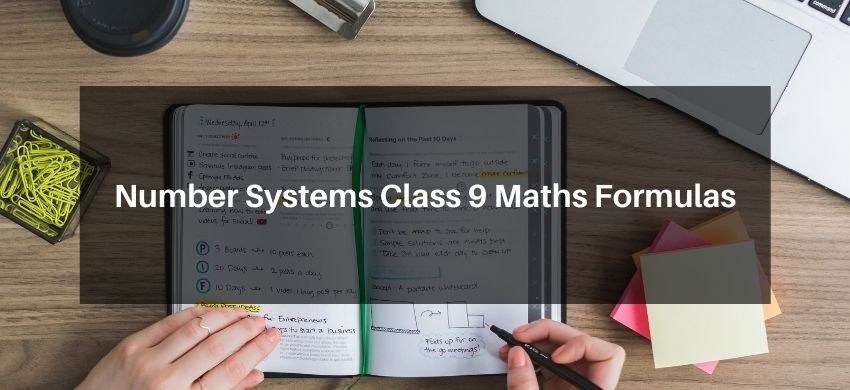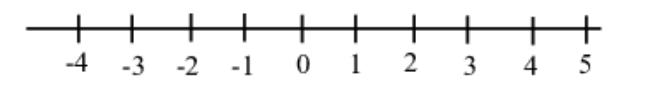Download the eSaral app and start learning from Kota's top IITians and doctors.

# Number Systems Class 9 Maths FormulasHey, students are you looking for Number Systems Class 9 Maths Formulas? If yes. Then you are at the right place. In this post, I have listed all the formulas of Number Systems class 9 that you can use to learn and understand the concepts easily.

If you want to improve your class 9 Math, Number Systems concepts, then it is super important for you to learn and understand all the formulas.

By using these formulas you will learn about the Number Systems.

With the help of these formulas, you can revise the entire chapter easily.

## Number Systems Class 9 Maths Formulas

• Natural numbers- Counting numbers started by 1 are called "Natural numbers" The set of natural numbers is denoted as $N=\{1,2,3, \ldots \ldots \ldots \ldots \ldots \infty\}$
• Whole Numbers- Natural numbers, including " 0 " (zero) are called whole numbers The set of whole numbers is $\mathrm{W}=\{0,1,2,3, \ldots \ldots \ldots\}$
• Integers- Whole numbers, including natural numbers with negative sign are called "Integers" The set of integers is $I$ or $Z=\{\ldots \ldots \ldots-3,-2,-1,0,1,2,3 \ldots \ldots \ldots \ldots\}$

(a) Odd numbers $-1,3,5,7, \ldots \ldots \ldots . \ldots$

(b) Even numbers- $0,2,4,6, \ldots \ldots \ldots . .$

(c) Prime number- Number divisible by one and itself only $2,3,5,7,11,13,17 \ldots .$
• Rational Numbers- Number of the form $p / q$ where $p, q \in I$ and $q \neq 0$ are called rational numbers. $Q=\left\{\frac{p}{q}, p \& q\right.$ are integers \& $\left.q \neq 0\right\}$
• Irrational numbers - Numbers which are non-terminating, non-recurring are called irrational numbers. Example- $\sqrt{2}, \sqrt{3}, \sqrt{7}$

The most popular irrational numbers

(i) $\quad \pi=3.1459265 \ldots \ldots \ldots \ldots .$

(ii) $\quad e=2.7182818$.
• Real numbers- Numbers including rational and irrational numbers are called "real numbers" Representation of real numbers on numbers line.Each and every point on the line represents a definite real number Each and every real number has definite chair on number line. All real numbers can be represented on a number line.